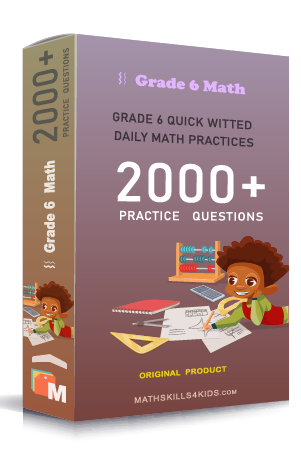# Grade 6 Fraction and mixed numbers worksheets with solutions

Subject
Math

Resource Type
Worksheets, Printables, Homeschool

Format
PDF (18.7 MB | 32 pages → Exercises + Solutions)

• ### -15% OFF Over \$100

Promo Code: TOPLA

After Purchase

\$3.25

This package allows you to practice the following skills

Solving fractions on number lines

Solving fractions of a whole word problems

Solving fractions of a group word problems

Finding equivalent fractions

Finding the least common denominator of fractions

Comparing fractions with like and unlike denominator

Comparing fractions word problems

converting between improper fractions and mixed numbers

Converting fractions to decimal numbers

Converting decimals to fractions

Putting a mix of decimals and fractions in order

Understanding fractions as division word problems

This product is a part of the Mega Pack
* Grade 6 quick-witted daily math practices *